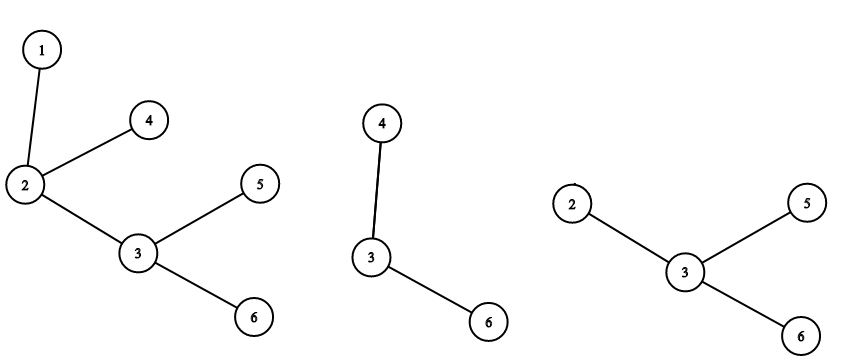시간 제한 메모리 제한 제출 정답 맞은 사람 정답 비율
2 초 256 MB 4 4 4 100.000%

## 문제

For a tree $T$ consisting of $n$ vertices numbered from $1$ to $n$, the compressed spanning subtree $S(X)$ of a set $X$ of spanned vertices (vertices that are not in $X$ are called not spanned) can be defined by the following algorithm:

1. Assign $S(X) \leftarrow T$;
2. If there is any not spanned vertex that has exactly one edge incident to it, remove it along with the edge;
3. Repeat step $2$ while its condition stays true;
4. If there is any not spanned vertex that has exactly two edges incident to it, remove it along with the edges and add a new edge connecting the two remaining endpoints of the removed edges;
5. Repeat step $4$ while its condition stays true.

Formally, $S(X)$ is the smallest subgraph of $T$ containing all vertices in $X$ and then having all other vertices of degree two or less smoothed out.The tree from test $1$, and its compressed spanning subtrees for $X = {3, 4, 6}$ and for $X = {2, 5, 6}$.

You are not given the tree $T$. Instead, your task is to find it. You can ask questions of the following form: "How many vertices does the compressed spanning subtree of $X$ contain?". And since otherwise finding the tree by asking such questions would be impossible, there are no vertices incident to exactly two edges in $T$.

## 프로토콜

The first line of input contains a single integer $n$ ($2 \le n \le 100$).

Your program can ask a question by printing a line in the format "? $k$ $x_1$ $x_2$ $\ldots$ $x_k$" where integer $k$ ($1 \le k \le n$) equals to the number of vertices in $X$ and distinct integers $x_i$ ($1 \le x_i \le n$) represent these vertices in any order. You can ask no more than $2550$ questions.

The answer for such question is an integer given on a separate line: the number of vertices in the compressed spanning subtree in question.

After asking sufficient questions, your program must give the answer by printing a line in the format "! $p_2$ $p_3$ $\ldots$ $p_n$". Here, considering $T$ as a rooted tree with root at vertex $1$, $p_i$ must be the parent vertex of vertex $i$. After giving the answer, the program must immediately terminate gracefully.

## 예제 입력 1

6

3

4

4



## 예제 출력 1


? 3 4 3 6

? 4 1 2 3 6

? 3 2 5 6

! 1 2 2 3 3


## 채점 및 기타 정보

• 예제는 채점하지 않는다.